#Quality Digest

Featured Product
This Week in Quality Digest Live
Statistics Features
Donald J. Wheeler
No one really understands kurtosis. Here’s why.
Alan Metzel
Introducing the Enhanced Perkin Tracker
Donald J. Wheeler
What you think you know may not be so
Tony Boobier
Why data leaders need to master words as well as statistics
Donald J. Wheeler
What they forgot to tell you in your statistics class
Statistics News
New capability delivers deeper productivity insights to help manufacturers meet labor challenges
Day and a half workshop to learn, retain, and transfer GD&T knowledge across an organization
Elsmar Cove is a leading forum for quality and standards compliance
InfinityQS’ quality solutions have helped cold food and beverage manufacturers around the world optimize quality and safety
User friendly graphical user interface makes the R-based statistical engine easily accessible to anyone
Collect measurements, visual defect information, simple Go/No-Go situations from any online device
Good quality is adding an average of 11 percent to organizations’ revenue growth
Ability to subscribe with single-user minimum, floating license, and no long-term commitment
A guide for practitioners and managersBio

Statistics

## What Are the Variance and Standard Deviation?

### And what do they tell us about our histogram?

Published: Monday, August 2, 2021 - 11:03

Your software routinely gives you four descriptive statistics for your data: the average, the standard deviation, the skewness, and the kurtosis. Of these only the average is easy to understand. This article and the next illustrate what these statistics are telling you about your data.

Welcome to Statistics Summer Camp where we use building blocks to create digital distributions. With these distributions we can discover what the various statistics do, and do not, tell us about our data.

### The average

When we compute an average we are creating a first-order simplification of the data. We are reducing them down to one characteristic. The average is that single value where we could place all of the data without changing the location of the data set as a whole. Thus, the average may be thought of as the balance point for the data. Of course, the data are not all equal to the average, and we do not usually draw a graph with all the data at the average, but for the purposes of describing our data, the average provides a first-order simplification of the data.

Our first example will consist of 24 values with an average of 9.000.

{ 5, 6, 6, 7, 7, 8, 8, 8, 8, 8, 9, 9,
9, 9, 9, 9, 11, 11, 11, 11, 11, 11, 12, 13 }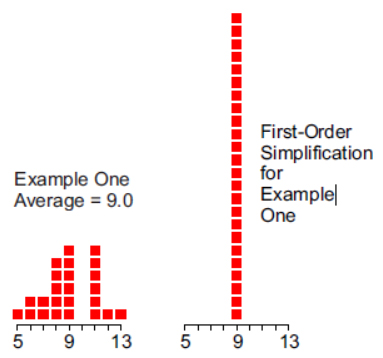Figure 1: The average as a first-order simplification for example one

As may be seen in figure 1, the location alone tells us nothing about how the data are spread out around the balance point.

### The standard deviation and variance

After the average, the second descriptive statistic is generally the standard deviation. Students are taught that this value defines dispersion, but how this works is seldom explained. Here, because we are interested in description rather than the estimation of an unknown parameter, we shall work with the “standard deviation” that is divided by [n] rather than [n-1]. The common name for this statistic is the root mean square deviation (RMSD).

Example one has an RMSD of 2.000 units. The “variance” statistic we shall use is the mean square deviation (MSD) which is 4.000 square units.

If we construct a second histogram with 12 values at [average – RMSD] = 7.000 and 12 values at [average + RMSD] = 11.000, this second histogram will also have an average of 9.0 and a RMSD of 2.000. Thus, the two histograms in figure 2 are equivalent in terms of location and dispersion. The histogram on the right is the second-order simplification for example one.Figure 2: The second-order simplification for example one

If we spin the second-order simplification about its balance point we would inscribe a circle on the x-plane with area equal to 4.000 times pi. The MSD for both of the histograms in figure 2 is 4.000 square units, and now you see why the variance is always expressed as an area.

Thus, the variance for a data set or a probability model is a property of the second-order simplification of that data set or probability model. When we spin the second-order simplification about the average, the mean square deviation is the area of the inscribed circle divided by pi. Those familiar with physics may recognize the MSD as the rotational inertia of the histogram.

The square root of rotational inertia divided by mass is the radius of gyration. In probability theory the mass is always equal to 1.00, so this means that the RMSD is the radius of gyration for the histogram.

Since, like gravity, you cannot cheat on rotational inertia, we can use the properties of rotational inertia to tell us more about the histogram of the data.

So the second-order simplification preserves both the balance point and the rotational inertia of the original histogram. It also defines regions for the original histogram. The region between the two spikes (from 7 to 11 in figure 2) defines the central portion of the histogram. Points outside the central portion are said to be in the tails of the histogram.

### Example two

Our second example will consist of 24 values with an average of 9.00 and an RMSD of 5.017.

{ 0, 1, 2, 4, 4, 4, 5, 5, 5, 5, 9, 11,
12, 12, 12, 13, 13, 13, 13, 13, 13, 13, 17, 17 }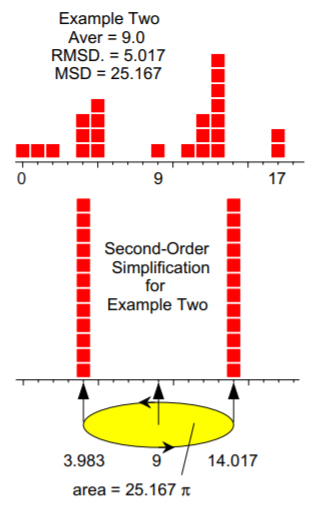Figure 3: Second-order simplification for example two

Thus, the variance (MSD) directly measures the rotational inertia of your data set, and the standard deviation (RMSD) gives the radius of gyration for the data about the average. The central portion of the histogram for example two extends from 4 to 14 and five values are found in the tails of the histogram.

To examine what rotational inertia can tell us about our histograms we will need some larger examples.

### Four digital models

Next we shall use our building blocks to construct some digital models that will mimic various continuous probability models. We use these digital models simply because our data are always digital, and these digital models will look and behave like histograms of data. By using these digital models we can begin to see some of the differences between the digital world in which we live and the mathematical world of continuous probability models.

The procedure for creating these digital models is explained in the appendix.

### A digital standard normal

Our first digital probability model will be based on the standard normal distribution. While the standard normal uses a continuous variable, our digital model will use 200 building blocks, each of which is 0.10 standard deviations wide.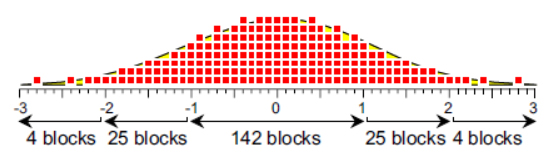Figure 4: A digital standard normal model

Due to the roundoff inherent in using the building blocks, our digital model is not as smooth as the standard normal distribution shown in the background. However, when using 200 blocks, this is as close as we can get to the continuous probability model. The block size of 0.10 standard deviations restricts where the blocks may be placed. Each of the blocks is centered as close as possible to the z-score for the median of each interval containing 0.005 of the area under the standard normal distribution.

### Two digital chi-square models

A standardized 8-degree-of-freedom chi-square distribution is shown by the smooth curve in figure 5. In the manner described in the appendix, using 200 blocks with a measurement increment of 0.1, we can find the digital version of this probability model shown in figure 5. Each block is centered as close as possible to the x-value for the median of each 0.005 of area under the standardized 8-degree-of-freedom chi-square distribution.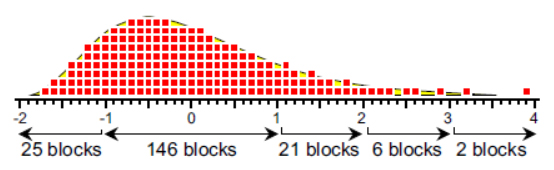Figure 5: A digital standardized 8 d.f. chi-square model

The standardized 4-degree-of-freedom chi-square distribution is shown in figure 6. Using 200 blocks with a measurement increment of 0.1 we can find the digital version of this probability model shown in figure 6. Each block is centered as close as possible to the x-value for the median of each 0.005 of area under the standardized 4-degree-of-freedom chi-square distribution.Figure 6: A digital standardized 4 d.f. chi-square model

### A digital exponential model

A standardized exponential distribution is shown by the smooth curve in figure 7. Using 200 blocks with a measurement increment of 0.1 we can find the digital version of this probability model shown in figure 7. Each block is centered as close as possible to the x-value for the median of each 0.005 of area under the standardized exponential distribution.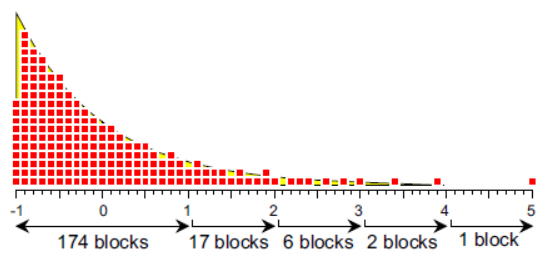Figure 7: A digital standardized exponential model

### Comparing the digital models

Even though these models have different shapes, they all have a mean of zero and a variance of one. As the upper tail gets elongated we see two things happening in the digital models. In order to maintain a variance of one we find an increasing number of blocks falling closer to the average. And in order to keep the average at zero we see the bulk of the blocks shifting over to the left side to balance the elongated tail on the right.

These two shifts result in an increasing number of blocks in the central portion of the model as the skewness of the model increases. This may be seen in the first column of figure 8. This increase in the central portion is the unavoidable consequence of having a few blocks further out in the extreme tail of the model. Balance and rotational inertia have to be preserved. Each point that gets moved out into the extreme tail requires that many more points get shifted closer to the average to maintain the average at zero and the MSD at 1.00.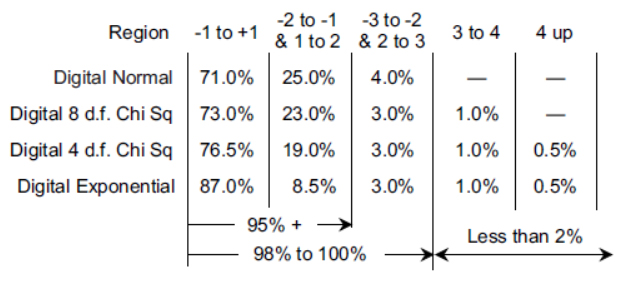Figure 8: Percentages of blocks in regions of the digital models

The second column of figure 8 summarizes the number of blocks found in the intervals from –2.0 to –1.1 and from 1.1 to 2.0. These intervals contain those blocks in the tails that are less than or equal to two standard deviations away from the average. As the skewness of the model increases the percentage of blocks in these intervals drop. This region is the primary source for the increased numbers of blocks in the central regions.

As a result, when we combine the first two columns we see that 95 percent to 96 percent of the blocks are found within two standard deviations of the average regardless of the skewness of the model. This characteristic of all mound-shaped probability models is a consequence of rotational inertia.

Rotational inertia requires at least 95 percent of the area to fall within two standard deviations of the mean for unimodal probability distributions having at least one infinite tail.

Another surprising characteristic of all mound-shaped probability models is found when we combine the first three columns in figure 8. Regardless of skewness, at least 98 percent of the area will fall within three standard deviations of the average.

### What about the elongated tails?

If our probability models are going to have at least 98 percent of their area within three standard deviations of the mean, then there will be at most 2 percent of the area in the extreme outer tails. As the skewness increases the tails do not get heavier, they simply become more attenuated. Approximately 99 percent of the area will remain within three standard deviations of the average as the extreme tails stretch the last 1 percent or 2 percent out ever more thinly. (For more rigorous treatments of this topic see “Properties of Probability Models,” Part 1, Part 2, and Part 3Quality Digest, August 3, September 1, and October 5, 2015.)

### Summary

So, once we know the average and the standard deviation statistic, we know several things about our data set. In the discussion above we were looking at probability models and their digital analogs. When we make allowance for the uncertainties introduced by working with data the percentages within each region become slightly softer. This is the origin of the empirical rule.

Once you have computed the average and standard deviation statistic for your data you can expect:

About 60 percent to 75 percent of the data within one standard deviation of the average.

Usually 90 percent to 98 percent of the data within two standard deviations of the average.

Approximately 99 percent to 100 percent of the data within three standard deviations of the average.

Given that we can say so much based on the first two statistics, what can the “shape statistics” of skewness and kurtosis add to the picture? That will be the topic of next month’s column.

A caveat is needed here. Data are historical. All descriptive statistics describe the past. Before the past may be used as a guide for the future your data will need to have come from a process that is being operated predictably. And to determine if this is the case you will have to use a process behavior chart.

Appendix: Creating digital models

To create a digital model for a given continuous probability distribution begin by choosing how many blocks you are going to use. Here we used 200 blocks so each block represented 1/200 = 0.005 of the area under the continuous probability model.

To find the location for the blocks we need to find the cumulative probabilities that correspond to the mid-points of each interval of 0.005 of cumulative probability. Starting with a cumulative probability of 0.0025, successively increase these cumulative probabilities by 0.005 until you reach the value of 0.9975.

Find the 200 points on the X-axis that correspond to each of these cumulative probabilities.

Round these X-values off to the measurement increment. Here the increment was 0.1.

Stack up the blocks at the resulting X-values.

For the standard normal distribution, a cumulative probability of 0.0025 defines the median of the first block of area 0.005. The cumulative probability of 0.0025 corresponds to a z-score of -2.81. A cumulative probability of 0.0075 corresponds to a z-score of -2.43. A cumulative probability of 0.0125 corresponds to a z-score of -2.24, etc. When rounded to one decimal place these values become -2.8, -2.4, and -2.2, etc.

Why use only one decimal place? With two decimal places we would have 601 possible values between -3.00 and +3.00, and our 200 points would be spread out in a thin line. To see the values mound up we need to round the z-scores off to one decimal place. These rounded z-scores tell us where to place the 200 blocks to get the digital standard normal distribution shown in figure 4.

### Discuss